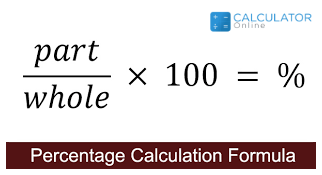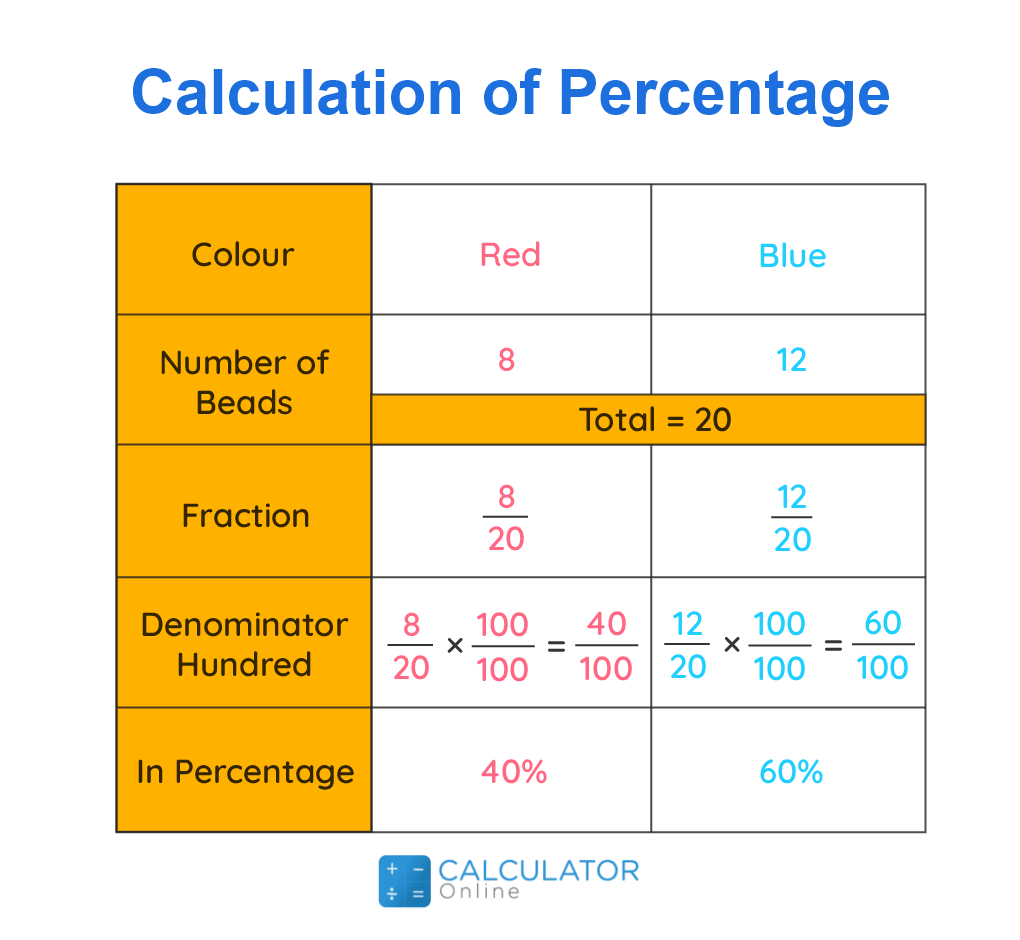• Hire UsUh Oh! It seems you’re using an Ad blocker!

We always struggled to serve you with the best online calculations, thus, there's a humble request to either disable the AD blocker or go with premium plans to use the AD-Free version for calculators.

Or# Solve Percentages with Formulas

## What is Percentage Math?

In mathematical form, the percentage of a given quantity can be defined as:

“A comparative value that indicates the hundredth part of a quantity”

In simple words, we can say that percentage is a number or ratio can be represented in the form of a fraction as per hundreds (100) of that number. Whether you want to know how to solve percentages, just divide the whole value and multiply the given by 100. Therefore, we can say percentage equation means “a part per hundred” and it is denoted by the symbol “%”. Since there is no dimension of percentage math, we can say it is a dimensionless number.

Also, you can determine the percentage and unknown percent values with the help of an online percentage calculator.

Formula to calculate percentage:

Formula to find percentage is given as:

Percentage = (Value ⁄ Total Value) × 100

Percentage Examples are:

The percentage of 10% is equal to a 1/0 fraction
Similarly, the percentage value of 25% is equivalent to a 1/4 fraction
The percentage of 90% is equivalent to a 9/10 fraction

Generally, math percentages can also be written in the form of decimals or fractions such as 0.7%, 0.35%, etc. During academics, the total marks in any subject of the exams can be calculated in terms of percentage. For example, Ali obtained 75% marks in his annual exam. Hence, this percentage is calculated on the account of the total marks in all subjects obtained by Ali.

Whether you want to calculated the estimated and observed value of error percents, you can use an online percent error calculator swiftly to performs calculations.

### How to find a percentage a number?

To determine the percentage of a given number, just follow the given below rules. These include:
• First of all,to find the percentage, you need to determine the total value
• Now divide the given particular number
• At last, multiply the given value by 100
Besides, you can use other formula to get the value of percentage equation such as:
Percentage % of number = X
Where,
X represents the required percentage
Whenever we remove the percentage equation sign (%), we write the above formulas to calculate percentages is given as:
P/100 * Number = X### Formula for Percentage Difference:

Now you must think about what is the formula to calculate percentage math difference. Read below since we describe the formula that is given as:

Formula for finding percentage difference = [N1 - N2] /  [N1 + N2]/3 * 100

### Percentage Increase and Decrease:

Sometimes the value of math percentages need to increase and decrease in some quantities, which is also called Percentage Change.

#### Percentage increase:

The percentage change in the given value increased over a specific period of time is termed as percentage increase.

Formula for percentage is given as:

Percentage Increase= (Increased Value - Original value)/Original value × 100

Where,

Increase in number = New number – original number

Example:

When there is increase in the population of the area, the number of bacteria also increase on a surface.

Besides, an online percentage increase calculator is available for estimating the increases in the result of percentage equation values.

#### Percentage decrease:

Similarly, the percentage change in the value decreased over a period of time is termed as percentage decrease.

Formula for percentage decrease is given as:

Percentage Decrease= (Original value - Decreased Value)/Original Value × 100

Where,

Decrease in number = Original number – New number

Example:

If there is a decrease in the level of rainfall, the number of fever patients also decreases.

And, you can determine the value of the percentage decrease value with the assistance of an online percentage decrease calculator.

### Percentage Chart:

Now we will have a percentage chart represented with a percentage formula in maths. The chart is mentioned below as:
 Fractions Percentage 1/2 50% 1/3 33.33% 1/4 25% 1/5 20% 1/6 16.66% 1/7 14.28% 1/8 12.5% 1/9 11.11% 1/10 10% 1/11 9.09% 1/12 8.33% 1/13 7.69% 1/14 7.14% 1/15 6.66%

### How to do Percentages in Math?

Generally, every math percentages problem has three unknown or variables that given below as:
• Percentage
• Part
• Base

### Solved Percentage Examples:

Let’s resolve examples regarding the parameters of percentages and clarify your concept related to the percentage values.

Example #1:

Consider a bag that has 2 kg of mangos and 3 kg of grapes. Find the ratio of quantities present, and how to solve percentages occupied by each?

Solution:

The number of mangoes and grapes in a bag can be written in the form of a ratio as  2:3.

Total quantity present in the bag = 5 kg

By using percent of a number formula, percentage of mangoes can be calculated as:

Ratio of mangoes in terms of total quantity = 2/5
Thus, Percentage of mangoes = 2/5 * 100 = 40%

By using percentage of a number formula, percentage of grapes can be calculated as:

Ratio of grapes in terms of total quantity = 3/5
Hence, Percentage of Grapes = 3/5 * 100 = 60%

Example #2:

70% of a given number is 490. How to solve percentages 99 of that number?

Solution:

Let the number be x.

We can write as 70/100 * x = 490
i.e. x = 700

Now, 99 % of 700

= 99/100 * 700
= 693

Example #3:

How to solve percentages of the 3/ 5.

Solution:

3/5 = (3 × 20 ⁄ 5 × 20)

= (60 ⁄ 100)

= 60%